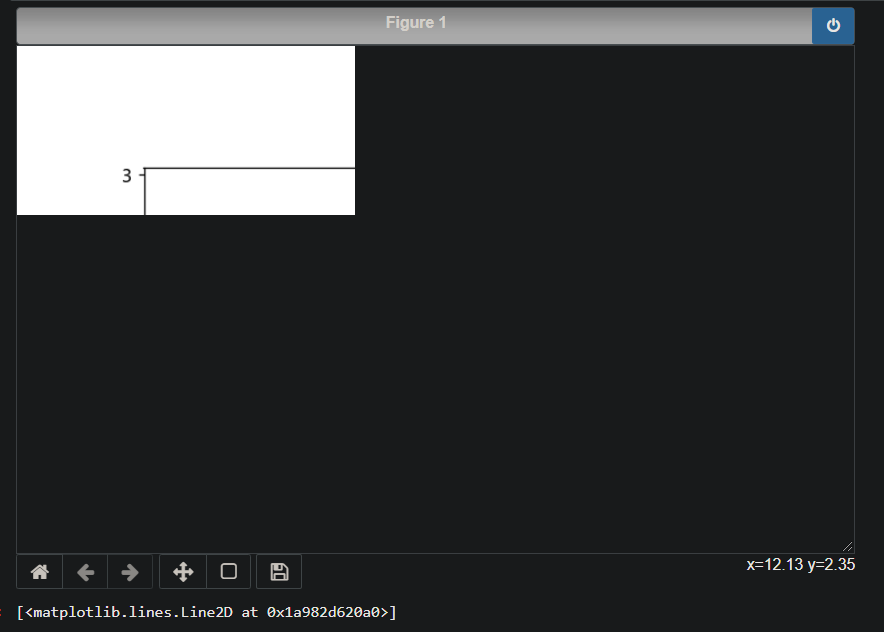2020-08-10 20:18

# Jupyter 使用%matplotlib notebook 进行交互式绘图时异常显示?

``````%matplotlib notebook
import matplotlib.pyplot as plt
import numpy as np

plt.plot(np.random.randn(50).cumsum())
``````• 点赞
• 写回答
• 关注问题
• 收藏
• 邀请回答